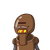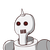# 15. Use Euclid division lemma to show that the square of any positive integer cannot be ofthe form 5m 2 or 5m – 3 for some i

15. Use Euclid division lemma to show that the square of any positive integer cannot be of
the form 5m 2 or 5m – 3 for some integer m. (3)​

### 2 thoughts on “15. Use Euclid division lemma to show that the square of any positive integer cannot be of<br />the form 5m 2 or 5m – 3 for some i”

1.Step-by-step explanation:

Hope it helps you ❣️❣️❣️❣️

2.Let a be any positive integer

By Euclid’s division lemma

a=bm+r

a=5m+r (when b=5)

So, r can be 0,1,2,3,4

Case 1:

∴a=5m (when r=0)

a

2

=25m

2

a

2

=5(5m

2

)=5q

where q=5m

2

Case 2:

when r=1

a=5m+1

a

2

=(5m+1)

2

=25m

2

+10m+1

a

2

=5(5m

2

+2m)+1

=5q+1 where q=5m

2

+2m

Case 3:

a=5m+2

a

2

=25m

2

+20m+4

a

2

=5(5m

2

+4m)+4

5q+4

where q=5m

2

+4m

Case 4:

a=5m+3

a

2

=25m

2

+30m+9

=25m

2

+30m+5+4

=5(5m

2

+6m+1)+4

=5q+4

where q=5m

2

+6m+1

Case 5:

a=5m+4

a

2

=25m

2

+40m+16=25m

2

+40m+15+1

=5(5m

2

+8m+3)+1

=5q+1 where q=5m

2

+8m+3

From these cases, we see that square of any positive no can’t be of the form 5q+2,5q+3.